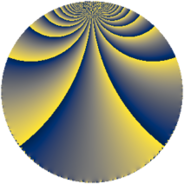# Properties

 Label 115.3.hLevel $115$ Weight $3$ Character orbit 115.h Rep. character $\chi_{115}(11,\cdot)$ Character field $\Q(\zeta_{22})$ Dimension $160$ Newform subspaces $1$ Sturm bound $36$ Trace bound $0$

# Related objects

## Defining parameters

 Level: $$N$$ $$=$$ $$115 = 5 \cdot 23$$ Weight: $$k$$ $$=$$ $$3$$ Character orbit: $$[\chi]$$ $$=$$ 115.h (of order $$22$$ and degree $$10$$) Character conductor: $$\operatorname{cond}(\chi)$$ $$=$$ $$23$$ Character field: $$\Q(\zeta_{22})$$ Newform subspaces: $$1$$ Sturm bound: $$36$$ Trace bound: $$0$$

## Dimensions

The following table gives the dimensions of various subspaces of $$M_{3}(115, [\chi])$$.

Total New Old
Modular forms 260 160 100
Cusp forms 220 160 60
Eisenstein series 40 0 40

## Trace form

 $$160 q + 4 q^{2} - 16 q^{4} - 26 q^{6} - 22 q^{8} - 32 q^{9} + O(q^{10})$$ $$160 q + 4 q^{2} - 16 q^{4} - 26 q^{6} - 22 q^{8} - 32 q^{9} + 30 q^{12} + 12 q^{13} - 256 q^{16} - 110 q^{17} + 70 q^{18} - 66 q^{19} - 66 q^{21} - 34 q^{23} + 180 q^{24} + 80 q^{25} + 238 q^{26} + 234 q^{27} + 128 q^{29} + 188 q^{31} + 496 q^{32} - 242 q^{34} - 170 q^{35} - 736 q^{36} - 770 q^{38} - 188 q^{39} - 440 q^{40} - 234 q^{41} - 176 q^{43} - 22 q^{44} + 80 q^{46} - 224 q^{47} + 754 q^{48} + 518 q^{49} + 90 q^{50} + 528 q^{51} - 82 q^{52} + 352 q^{53} + 510 q^{54} + 400 q^{55} + 418 q^{56} - 726 q^{57} + 376 q^{58} - 62 q^{59} + 330 q^{60} - 308 q^{61} - 662 q^{62} - 550 q^{63} - 206 q^{64} - 176 q^{66} - 44 q^{67} - 280 q^{69} - 120 q^{70} - 18 q^{71} + 1126 q^{72} + 52 q^{73} + 154 q^{74} + 704 q^{76} - 726 q^{77} - 1434 q^{78} - 572 q^{79} + 476 q^{81} + 46 q^{82} + 286 q^{83} - 1100 q^{84} - 130 q^{85} + 396 q^{86} - 1012 q^{87} - 528 q^{88} - 264 q^{89} + 350 q^{92} + 604 q^{93} + 444 q^{94} - 80 q^{95} - 394 q^{96} + 792 q^{97} + 540 q^{98} + 2112 q^{99} + O(q^{100})$$

## Decomposition of $$S_{3}^{\mathrm{new}}(115, [\chi])$$ into newform subspaces

Label Dim $A$ Field CM Traces $q$-expansion
$a_{2}$ $a_{3}$ $a_{5}$ $a_{7}$
115.3.h.a $160$ $3.134$ None $$4$$ $$0$$ $$0$$ $$0$$

## Decomposition of $$S_{3}^{\mathrm{old}}(115, [\chi])$$ into lower level spaces

$$S_{3}^{\mathrm{old}}(115, [\chi]) \cong$$ $$S_{3}^{\mathrm{new}}(23, [\chi])$$$$^{\oplus 2}$$